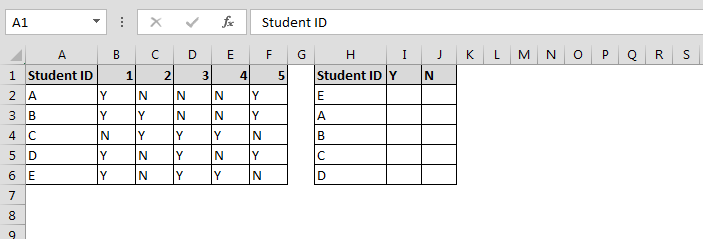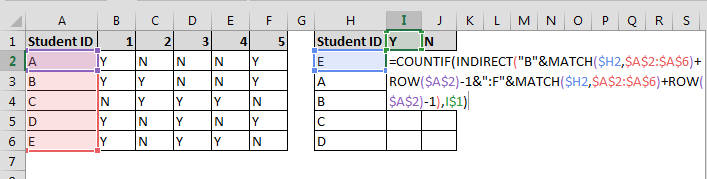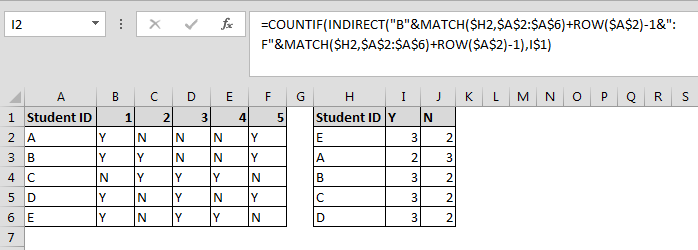Creating a Summary Table of a Student Questionnaire

In this chapter, you’ll learn about how to create a summary table of a student questionnaire in Microsoft Excel by using the functions. We will make use of COUNTIF, MATCH, INDIRECT and ROW functions.

Let’s take an example and understand how all these formulas will work:-

Column A contains student name, columns B to F contains the answer (Y/N) to 5 questions from each of the 5 students.

Each row includes the answers of one of the student. We want to create a summary table that will show a count of the questions answered Y and N for each student.• Enter the formula in cell I2
• =COUNTIF(INDIRECT("B"&MATCH(\$H2,\$A\$2:\$A\$6)+ROW(\$A\$2)-1&":F"&MATCH(\$H2,\$A\$2:\$A\$6)+ROW(\$A\$2)-1),I\$1)• Press Enter
• Copy the same in the range I2:J6As you can see in the above image according to Student ID function will count the number of replies as per the categories.

Formula Explanation:-

• We have used COUNTIF function to count the Y/N category numbers
• Indirect function we have used to provide the range to COUNTIF function
• Match function we have used to match the categories and student id
• Row function is also used to arrange the range value